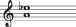##### Music Theory For DummiesYou can use music theory to build intervals. The first step in building any interval when creating a piece of music is to create the desired quantity, or number size, above or below a given note. Then you determine the quality.

## Determining quantity

Determining quantity is easy, especially on paper. For, say, a unison interval, just pick a note. Then, right next to the first note, put another one exactly like it.

Want to make your interval an octave? Put the second note exactly seven lines and spaces above or below the first, making for a total interval quantity of eight lines and spaces.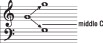How about a fourth? Put the second note three spaces and lines above or below the first note, making for a total interval quantity of four lines and spaces. And a fifth? Put the second note four spaces and lines above or below the first note, making for a total interval quantity of five lines and spaces.

## Establishing the quality

The second step to building an interval is to decide what the quality of the interval will be. Say, for example, your starting note is an A flat. And suppose you want your interval to be a perfect fifth above A flat. First, you count out the quantity needed for the fifth interval, meaning you count an additional four spaces above the starting note, making for a total quantity of five lines and spaces.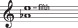Next, you have to alter the second note in order to make it a perfect fifth. Because all fifths are perfect so long as both notes have the same accidental (except for darned B and F), in order to make this pair a perfect fifth, you have to flat the second note so it matches the first.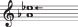If you want to make the second note an augmented fifth (abbreviated aug5 or A5) below A, you count down an additional four lines and spaces from A to make a total quality of five lines and spaces, and then you write the note, which is D.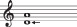Now you alter your added note to make the interval augmented. As you now know, a fifth is an augmented fifth when an additional half step is added to the interval (7 + 1 = 8 half steps), so you would lower the bottom note to a D flat.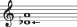To make the second note a diminished fifth (abbreviated dim5 or D5) above A, you count up an additional four lines and spaces, to make a total quality of five lines and spaces, and then write the note, which is E.

Finally, you alter your added note to make the interval diminished. A fifth is a diminished fifth when a half step is removed from the perfect fifth (7 – 1 = 6 half steps), so you would lower the top note to an E flat. (Note that a diminished fifth is the same as an augmented fourth — both intervals are made up of six half steps.)